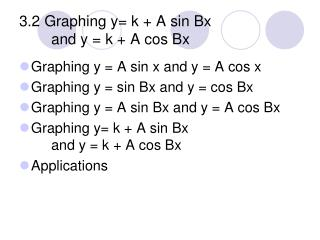Download Presentation3.2 Graphing y= k + A sin Bx and y = k + A cos Bx

# 3.2 Graphing y= k + A sin Bx and y = k + A cos Bx - PowerPoint PPT PresentationDownload Presentation## 3.2 Graphing y= k + A sin Bx and y = k + A cos Bx

- - - - - - - - - - - - - - - - - - - - - - - - - - - E N D - - - - - - - - - - - - - - - - - - - - - - - - - - -
##### Presentation Transcript

1. 3.2 Graphing y= k + A sin Bx and y = k + A cos Bx • Graphing y = A sin x and y = A cos x • Graphing y = sin Bx and y = cos Bx • Graphing y = A sin Bx and y = A cos Bx • Graphing y= k + A sin Bx and y = k + A cos Bx • Applications

2. Comparing Amplitudes • Compare the graphs of y = 1/3 sin x and y = 3 sin x • The effect of A in y = A sin x is to increase or decrease the y values without affecting the x values.

3. Comparing Periods • Compare the graphs of y = sin 2x and y = sin ½ x The graph shows the change in the period.

4. Amplitude and Period For both y = A sin Bx and y = A cos Bx: Amplitude = |A| Period = 2p/B

5. Vertical Shift • y = -2 + 3 cos 2x, -p x  2p • Find the period, amplitude, and phase shift and then graph

6. Period and Frequency • For any periodic phenomenon, if P is the period and f is the frequency, P = 1/f.